# JP4350750B2 - Retransmission control method and communication apparatus - Google Patents

## Info

Publication number
JP4350750B2
JP4350750B2 JP2006519135A JP2006519135A JP4350750B2 JP 4350750 B2 JP4350750 B2 JP 4350750B2 JP 2006519135 A JP2006519135 A JP 2006519135A JP 2006519135 A JP2006519135 A JP 2006519135A JP 4350750 B2 JP4350750 B2 JP 4350750B2
Authority
JP
Japan
Prior art keywords
matrix
time
check matrix
parity check
retransmission
Prior art date
Legal status (The legal status is an assumption and is not a legal conclusion. Google has not performed a legal analysis and makes no representation as to the accuracy of the status listed.)
Active
Application number
JP2006519135A
Other languages
Japanese (ja)
Other versions
JPWO2005107081A1 (en
Inventor

Original Assignee

Priority date (The priority date is an assumption and is not a legal conclusion. Google has not performed a legal analysis and makes no representation as to the accuracy of the date listed.)
Filing date
Publication date
Application filed by 三菱電機株式会社 filed Critical 三菱電機株式会社
Priority to PCT/JP2004/006137 priority Critical patent/WO2005107081A1/en
Publication of JPWO2005107081A1 publication Critical patent/JPWO2005107081A1/en
Application granted granted Critical
Publication of JP4350750B2 publication Critical patent/JP4350750B2/en
Application status is Active legal-status Critical
Anticipated expiration legal-status Critical

## Images

•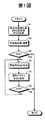••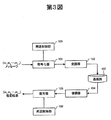•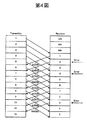•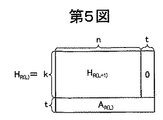•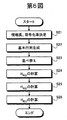•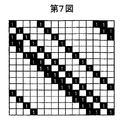•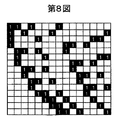•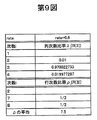••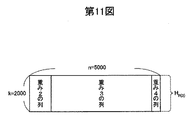•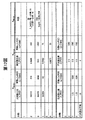•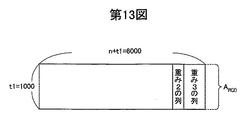•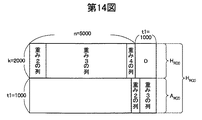•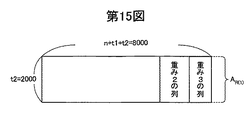•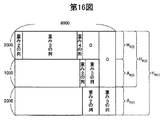•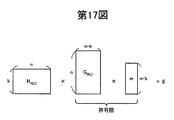•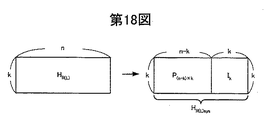•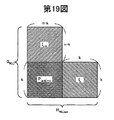•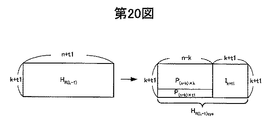•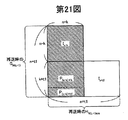•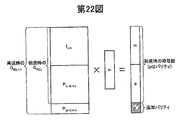## Classifications

• HELECTRICITY
• H03BASIC ELECTRONIC CIRCUITRY
• H03MCODING; DECODING; CODE CONVERSION IN GENERAL
• H03M13/00Coding, decoding or code conversion, for error detection or error correction; Coding theory basic assumptions; Coding bounds; Error probability evaluation methods; Channel models; Simulation or testing of codes
• H03M13/03Error detection or forward error correction by redundancy in data representation, i.e. code words containing more digits than the source words
• H03M13/05Error detection or forward error correction by redundancy in data representation, i.e. code words containing more digits than the source words using block codes, i.e. a predetermined number of check bits joined to a predetermined number of information bits
• H03M13/11Error detection or forward error correction by redundancy in data representation, i.e. code words containing more digits than the source words using block codes, i.e. a predetermined number of check bits joined to a predetermined number of information bits using multiple parity bits
• H03M13/1102Codes on graphs and decoding on graphs, e.g. low-density parity check [LDPC] codes
• H03M13/1148Structural properties of the code parity-check or generator matrix
• HELECTRICITY
• H03BASIC ELECTRONIC CIRCUITRY
• H03MCODING; DECODING; CODE CONVERSION IN GENERAL
• H03M13/00Coding, decoding or code conversion, for error detection or error correction; Coding theory basic assumptions; Coding bounds; Error probability evaluation methods; Channel models; Simulation or testing of codes
• H03M13/03Error detection or forward error correction by redundancy in data representation, i.e. code words containing more digits than the source words
• H03M13/05Error detection or forward error correction by redundancy in data representation, i.e. code words containing more digits than the source words using block codes, i.e. a predetermined number of check bits joined to a predetermined number of information bits
• H03M13/11Error detection or forward error correction by redundancy in data representation, i.e. code words containing more digits than the source words using block codes, i.e. a predetermined number of check bits joined to a predetermined number of information bits using multiple parity bits
• H03M13/1102Codes on graphs and decoding on graphs, e.g. low-density parity check [LDPC] codes
• H03M13/1148Structural properties of the code parity-check or generator matrix
• H03M13/1151Algebraically constructed LDPC codes, e.g. LDPC codes derived from Euclidean geometries [EG-LDPC codes]
• HELECTRICITY
• H03BASIC ELECTRONIC CIRCUITRY
• H03MCODING; DECODING; CODE CONVERSION IN GENERAL
• H03M13/00Coding, decoding or code conversion, for error detection or error correction; Coding theory basic assumptions; Coding bounds; Error probability evaluation methods; Channel models; Simulation or testing of codes
• H03M13/63Joint error correction and other techniques
• H03M13/6306Error control coding in combination with Automatic Repeat reQuest [ARQ] and diversity transmission, e.g. coding schemes for the multiple transmission of the same information or the transmission of incremental redundancy
• HELECTRICITY
• H03BASIC ELECTRONIC CIRCUITRY
• H03MCODING; DECODING; CODE CONVERSION IN GENERAL
• H03M13/00Coding, decoding or code conversion, for error detection or error correction; Coding theory basic assumptions; Coding bounds; Error probability evaluation methods; Channel models; Simulation or testing of codes
• H03M13/63Joint error correction and other techniques
• H03M13/635Error control coding in combination with rate matching
• H03M13/6362Error control coding in combination with rate matching by puncturing
• H03M13/6368Error control coding in combination with rate matching by puncturing using rate compatible puncturing or complementary puncturing
• H03M13/6393Rate compatible low-density parity check [LDPC] codes
• HELECTRICITY
• H04ELECTRIC COMMUNICATION TECHNIQUE
• H04LTRANSMISSION OF DIGITAL INFORMATION, e.g. TELEGRAPHIC COMMUNICATION
• H04L1/00Arrangements for detecting or preventing errors in the information received
• H04L1/004Arrangements for detecting or preventing errors in the information received by using forward error control
• H04L1/0056Systems characterized by the type of code used
• H04L1/0057Block codes
• HELECTRICITY
• H04ELECTRIC COMMUNICATION TECHNIQUE
• H04LTRANSMISSION OF DIGITAL INFORMATION, e.g. TELEGRAPHIC COMMUNICATION
• H04L1/00Arrangements for detecting or preventing errors in the information received
• H04L1/08Arrangements for detecting or preventing errors in the information received by repeating transmission, e.g. Verdan system
• HELECTRICITY
• H04ELECTRIC COMMUNICATION TECHNIQUE
• H04LTRANSMISSION OF DIGITAL INFORMATION, e.g. TELEGRAPHIC COMMUNICATION
• H04L1/00Arrangements for detecting or preventing errors in the information received
• H04L1/12Arrangements for detecting or preventing errors in the information received by using return channel
• H04L1/16Arrangements for detecting or preventing errors in the information received by using return channel in which the return channel carries supervisory signals, e.g. repetition request signals
• H04L1/18Automatic repetition systems, e.g. van Duuren system ; ARQ protocols
• H04L1/1812Hybrid protocols
• H04L1/1819Hybrid protocols with retransmission of additional or different redundancy
• HELECTRICITY
• H04ELECTRIC COMMUNICATION TECHNIQUE
• H04LTRANSMISSION OF DIGITAL INFORMATION, e.g. TELEGRAPHIC COMMUNICATION
• H04L1/00Arrangements for detecting or preventing errors in the information received
• H04L1/12Arrangements for detecting or preventing errors in the information received by using return channel
• H04L1/16Arrangements for detecting or preventing errors in the information received by using return channel in which the return channel carries supervisory signals, e.g. repetition request signals
• H04L1/1607Details of the supervisory signal

## Description

The present invention relates to a retransmission control method that can be realized in a system that employs a low-density parity-check (LDPC) code as an error correction code, and a communication apparatus that constitutes the system. The present invention relates to a retransmission control method and a communication apparatus when an LDPC code is applied to a Type-II HARQ (Hybrid Automatic Repeat reQuest).

Hereinafter, a conventional retransmission control method will be described. For example, error control includes error correction coding (FEC: Forward Error Correction) and automatic retransmission request (ARQ: Automatic Repeat reQuest), but in packet transmission, it is necessary to guarantee error-free transmission. Error control is essential. In particular, in a system in which the optimum modulation method and coding method are selected according to the propagation path state (adaptive modulation / demodulation / error correction) to improve the throughput, packet errors are unavoidable. An embedded HARQ scheme is required.
As the HARQ scheme, there are Type-I type HARQ in which the retransmission packet is the same as the original packet, and Type-II type HARQ in which the retransmission packet is different from the original packet.
Here, an example of the Type-II type HARQ will be described. The Type-II type HARQ basically transmits information bits at the time of initial transmission and transmits parity bits for error correction at the time of retransmission. Here, the Type-II type HARQ is taken as an example. A case where the present invention is applied to a system using a turbo code will be described (see Non-Patent Document 1). For example, in a system using a turbo code, a communication device on the transmission side encodes an information signal sequence at a coding rate R, and then encodes redundant bits (parity bits) based on a predetermined erasure rule. Decrease and send. At the time of retransmission, a packet composed of only additional parity, which is different from the packet at the time of initial transmission, is transmitted. On the other hand, the receiving side communication device code-synthesizes the received packet and the retransmitted packet stored in the receiving buffer at the time of initial transmission, and performs a decoding process with a smaller coding rate according to the number of retransmissions.
In Type-II HARQ, such a series of processing is repeatedly executed until no error is detected, thereby realizing error-free transmission and further improving reception characteristics by improving coding gain.
[Non-Patent Document 1]
J. et al. Xu, “Turbo Coded Hybrid Type II ARQ System” Master's thesis, Chalmers University of Technology, School of Electric and Computer Eng.
However, in the retransmission control method using the turbo code described in the above-mentioned document, there is a problem that the greater the number of bits to be erased, the farther the distance from the Shannon limit is, and the characteristics deteriorate. In addition, in the retransmission control method using the turbo code, even if additional parity is transmitted at the time of retransmission, it is not possible to determine whether the selected parity is the optimum parity, so the original performance of the turbo code may not be obtained. There was also a problem that there was.
The present invention has been made in view of the above, and provides a retransmission control method and a communication apparatus capable of always obtaining the original performance of an error correction code with stable characteristics in Type-II type HARQ. It is aimed.

The retransmission control method according to the present invention employs a low density parity check (LDPC) code as an error correction code, transmits a codeword after encoding at a predetermined coding rate at the time of initial transmission, and at the time of retransmission. A retransmission control method for a communication device on the transmission side that transmits additional parity, for example, a parity check matrix at the time of initial transmission optimized at a specific coding rate, and stepwise while lowering the coding rate A parity check matrix generating step for generating a parity check matrix at retransmission (arbitrary number of retransmissions), and an irreducible standard parity check matrix (check symbol generation matrix and unit) An initial irreducible standard form check matrix generation step for converting into a matrix)), and an initial irreducible standard form generation matrix for generating an initial irreducible standard form generation matrix including the check symbol generation matrix. Generation step and the initial transmission Generate a codeword using a generation matrix of approximately standard form and fixed-length information (m) and transmit the codeword, and generate the check matrix when a NAK is received from the communication device on the receiving side. Generating an additional parity based on a parity check matrix (corresponding to one of the parity check matrices at the time of retransmission) corresponding to a coding rate one step lower than the current coding rate generated in the step; A retransmission control step for transmitting, and thereafter, when a NAK is received, the retransmission control step is repeatedly executed while decreasing the coding rate by one step until an ACK is returned.
In the retransmission control method according to the present invention, for example, an LDPC code having excellent characteristics very close to the Shannon limit is applied as an error correction code when adopting Type-II type HARQ. Generate a generation matrix at retransmission from a parity check matrix corresponding to a coding rate lower than the coding rate at the time of initial transmission or the previous retransmission, and transmit only additional parity based on the generation result It was.

FIG. 1 is a flowchart showing a retransmission control method (processing of a communication device on the transmission side) according to the present invention, and FIG. 2 shows a retransmission control method (processing of a communication device on the reception side) according to the present invention. FIG. 3 is a diagram showing an LDPC encoding / decoding system, FIG. 4 is a diagram showing a Type-II type HARQ process, and FIG. 5 is a parity check matrix HR ( L) is a diagram showing the configuration of FIG. 6 is a flowchart showing a configuration method based on Euclidean geometry codes "Irregular-LDPC code", FIG. 7 is a Euclid geometry code EG (2, 2 2) FIG. 8 is a diagram showing a matrix after rearrangement, FIG. 9 is a diagram showing degree distribution after optimization calculation, and FIG. 10 is a diagram after adjustment. Diagram showing degree distribution There, FIG. 11 is a diagram illustrating a parity check matrix H R (3), FIG. 12 is a diagram showing the order allocation obtained as a result of the optimization calculation, FIG. 13, the additional matrix is a diagram showing an a R (2), FIG. 14 is a diagram illustrating a parity check matrix H R (2), FIG. 15 is a diagram showing an additional matrix a R (1), 16 FIG. 17 is a diagram illustrating a parity check matrix HR (1) , FIG. 17 is a diagram illustrating conditions for generating a generator matrix GR (L) , and FIG. 18 is an irreducible standard form. FIG. 19 is a diagram showing a conversion process to a parity check matrix H R (L) sys = [P (n−k) xk | I k ], and FIG. 19 shows an irreducible standard generation matrix G R at the time of initial transmission. FIG. 20 shows a generation process of (L) , and FIG. 20 shows an irreducible standard form check matrix HR (L−1) sys = [P (n−k) × (k + t1). | I k + t1 ], FIG. 21 is a diagram showing the generation process of the irreducible standard form generator matrix GR (L−1) at the time of retransmission, and FIG. It is a figure which shows the codeword at the time of resending.

In order to explain the present invention in more detail, it will be described with reference to the accompanying drawings.
1 and 2 are flowcharts showing a retransmission control method according to the present invention. Specifically, FIG. 1 shows processing of a communication device on the transmission side, and FIG. 2 shows processing of the communication device on the reception side. Indicates processing. Here, a retransmission control method when an LDPC code having excellent characteristics very close to the Shannon limit, for example, is applied as an error correction code when adopting Type-II type HARQ.
Note that the parity check matrix H for the LDPC code in this embodiment.R (L)May be generated in the communication device in accordance with a set parameter, or may be generated by another control device (computer or the like) outside the communication device. Parity check matrix HR (L)Is executed outside the communication device, the generated parity check matrix HR (L)Is stored in the communication device. In the following embodiments, the parity check matrix H in the communication deviceR (L)The case of generating will be described. Where R (L) represents a coding rate, and L = 1, 2, 3,..., Max (0 <R (1) <R (1) <... <R (max-1) <R (max ) = 1). R (max) means no encoding.
Here, before describing the retransmission control method of the present embodiment, first, the positioning of the encoder and the decoder capable of realizing the retransmission control method of the present embodiment will be described.
FIG. 3 is a diagram showing an LDPC encoding / decoding system. In FIG. 3, the transmission-side communication apparatus includes an encoder 101, a modulator 102, and a retransmission control unit 103, and the reception-side communication apparatus includes a demodulator 104, a decoder 105, and a retransmission control unit 106. Including configuration. Note that, here, for convenience of explanation, the configuration required on the transmission side (configuration of the transmitter) and the configuration required on the reception side (configuration of the receiver) are described separately. It is good also as providing both structures as a communication apparatus which can implement | achieve this communication.
In the encoder 101 on the transmission side, for example, a parity check matrix H for an LDPC code corresponding to a desired coding rate by a parity check matrix configuration method of the present embodiment described later.R (max-1)~ HR (1)Is generated. And, for example, at the time of initial transmission (coding rate: R (L)), the generator matrix G is based on the following conditions:R (L)Ask for.
GR (L): (N−k) × n matrix (n−k: information length, n: code length)
HR (L)× GR (L)= 0
Thereafter, the encoder 101 transmits a message (m1, M2, ..., mnk) And the generator matrix GR (L)Codeword C with code length n usingR (L)Is generated.
CR (L)= (M1, M2, ..., mnk) X GR (L)
= (C1, C2, ..., cn(However, HR (L)(C1, C2, ..., cn)T= 0)
Then, the modulator 102 generates the generated codeword C.R (L)On the other hand, digital modulation such as BPSK, QPSK, and multilevel QAM is performed and transmitted.
On the other hand, on the receiving side, the demodulator 104 performs digital demodulation such as BPSK, QPSK, and multilevel QAM on the modulated signal received via the communication path 107, and the decoder 105 is LDPC encoded. The demodulated result is iteratively decoded by the “sum-product algorithm”, and the estimation result (original m1, M2, ..., mnkIs output).
Next, the operation of each communication apparatus in the LDPC encoding / decoding system, that is, the retransmission control method in the present embodiment will be described in detail with reference to FIGS. In this embodiment, for convenience of explanation, retransmission control when focusing on one information sequence will be described. However, in Type-II type HARQ, as shown in FIG. Are transmitted continuously and retransmission control is performed when NAK (NAK # 2, NAK # 4, NAK # 8) is returned.
First, in the communication device on the transmission side, the encoder 101 is used for the LDPC code at the initial transmission based on a predetermined coding rate R (L) (L = 2 to max-1 at the initial transmission). Parity check matrix HR (L)(N × k matrix) is obtained, and further, the parity check matrix H for the LDPC code at the time of retransmission, at the time of retransmission,...R (L-1), HR (L-2),... Are obtained (FIG. 1, step 31). And then. Parity check matrix H at the first transmissionR (L)To “HR (L)× GR (L)= 0 ”R (L)((N−k) × n matrix) is obtained (step S1).
Here, a configuration method of the parity check matrix for the LDPC code in the encoder 101 will be described in detail. In the present embodiment, as an example, a method of constructing a parity check matrix for an Irregular-LDPC code based on Euclidean geometry (details of step S1 in FIG. 1) will be described.
Note that the parity check matrix HR (L)Is expressed by a general expression, a parity check matrix H whose coding rate is one step higherR (L + 1)And an additional parity check matrix AR (L)Can be defined as in the following formula (1). FIG. 5 is a diagram showing an outline of equation (1).
However, the parity check matrix HR (L)And parity check matrix HR (L + 1)Are both full rank (linearly independent).
In the present embodiment, the parity check matrix H is determined by the Gaussian approximation method.R (L), L = 1, 2,..., Max order distribution is optimized. That is, a parity check matrix H that minimizes the following equation (2):R (L)Find the order distribution of.
However, GAPR (L)Is a parity check matrix H estimated by the Gaussian approximation method.R (L)The difference between the SNR of the iteration threshold and the Shannon limit is expressed in dB.
Also, a parity check matrix H that minimizes the above equation (2).R (L)For example, the following equation (3), that is, Gaussian noise σnA calculation for searching for λ (x, R (L)), ρ (x, R (L)) that maximizes (R (L)) is performed. The following constraints (4), (5), (6), and (7) show the constraint conditions for calculating the following (3).
Where λi(R (L)) is a parity check matrix HR (L)Represents the ratio of columns of order ii(R (L)) is the parity check matrix HR(I)Represents the ratio of rows of order i. Also, dv (max, R (L)) is a parity check matrix HR (L)Dc (max, R (L)) is a parity check matrix HR (L)Represents the maximum degree of the row. Λ (x, R (L)) is a parity check matrix HR (L)Is the generation function of the degree distribution of the columns of ρ, and ρ (x, R (L)) is the parity check matrix HR (L)Is the generation function of the degree distribution of the rows of. N, (i, R (L)) is a parity check matrix HR (L)Represents the number of columns of order i, and nc(I, R (L)) is a parity check matrix HR (L)Represents the number of rows of order i.
Hereinafter, in step S1, the parity check matrix HR (L)As an example of a process for obtaining the parity check matrix HR (3), Parity check matrix HR (2), Parity check matrix HR (1)The processing for obtaining the values in order will be specifically described. FIG. 6 is a flowchart showing a configuration method of an “Irregular-LDPC code” based on the Euclidean geometric code.
First, the encoder 101 determines the information length and coding rate (FIG. 6, step S21). Here, for example, the information length is nk = 3000, and the coding rates are R (3) = 0.6, R (2) = 0.5, and R (1) = 0.375. In this case, the code length (information length / coding rate) at the time of initial transmission is n = 5000, the code length at the time of retransmission is n + t1 = 6000 (t1 = 1000), and the code length at the time of retransmission is n + t1 + t2 = 8000 ( t2 = 2000).
Next, in the encoder 101, the Euclidean geometric code EG (2, 2s), And the basic matrices A (s = 5, R (3)), A (s = 5, R (2)), A (, which are the bases of the parity check matrix for the “Irregular-LDPC code” s = 5, R (1)) is generated (step S22). For example, when s = 5, Euclidean geometric code EG (2, 25) In the first row (column number “1”) is as follows.
{1 32 114 136 149 223 260 382 402 438 467 507 574 579 588 622 634 637 638 676 717 728 790 851 861 879 947 954 971 977 979 998}
In encoding / decoding using an LDPC code, generally, the smaller the number of “cycle 4” and “cycle 6” on a bipartite graph, the better characteristics can be obtained. Therefore, in the present embodiment, the Euclidean geometric code EG (2, 2) is used so as to suppress a small number of cycles such as “cycle 4” and “cycle 6”.5) Is appropriately thinned out from the weight distribution in the first row. The weight distribution after thinning is, for example, as follows.
{1 32 114 136 149 223 260 260 402 438 467 507 574 588 634 638 717 728 790 861 947 971 979}
Based on the weight distribution after thinning, the weight distribution of the first row of each basic matrix is determined (individually assigned the position “1”), and the weight distribution is cyclically shifted to obtain 1023 rows. A basic matrix A (s = 5, R (3)), A (s = 5, R (2)), A (s = 5, R (1)) having × 1023 columns is generated. In the present embodiment, the weight distribution of the first row of each basic matrix is determined as follows, for example.
A (s = 5, R (3)) = {1 32 114 149 260 402 467 507 574 634 717 728 790 861 979}
A (s = 5, R (2)) = {223 438 947}
A (s = 5, R (1)) = {136 588 638 971}
Thereby, the parity check matrix HR (3)The maximum degree of the column of dv (max, R (3)) = 15, and the parity check matrix HR (2)The maximum degree of the column of dv (max, R (2)) = 3, and the parity check matrix HR (1)The maximum order of the column is dv (max, R (1)) = 4. In addition, the parity check matrix HR (3)The maximum degree of the row of dc (max, R (3)) = 15, and the parity check matrix HR (2)The maximum degree of the row of dc (max, R (2)) = 3, and the parity check matrix HR (1)The maximum order of the row is dc (max, R (1)) = 4.
Next, the encoder 101 rearranges each of the basic matrices according to the following procedure so that the position of “1” in the column is as high as possible in the column (step S23). If this rearrangement procedure is generally expressed, it can be expressed as the following equation (8).
I = 1 to 22s-1. Also, the polynomial (X(W1-1)+ X(W2-1)+ ...) is an expression representing the first row of each basic matrix. For example, when the position of the weight of the basic matrix is {179... 40}, 1 + X(7-1)+ X(9-1)+ ... X(40-1)It becomes.
In the above equation (8), i = 1 to 22s−1, j = 1 to i−1, hi(X) = hjIf (X) exists, hiDelete (X). With this rearrangement process, when performing a row deletion process (shortening process), which will be described later, it is possible to leave a column with as much weight as possible, and to reduce variations in the weight within the column as much as possible.
As a specific example, for example, Euclidean geometric code EG (2, 22) Is a basic matrix, the matrix shown in FIG. 7 is rearranged like the matrix shown in FIG. FIG. 7 shows the Euclidean geometric code EG (2, 22) Is a diagram illustrating a matrix (blank represents 0), and FIG. 8 is a diagram illustrating a matrix after rearrangement.
Next, in the encoder 101, the information length n−k = 3000 (code length n = 5000) determined above, the coding rate R (3) = 0.6, the rearranged basic matrix A (s = 5, R (3)), a parity check matrix H of n × k (5000 columns × 2000 rows)R (3)Is executed (optimization calculation) (step S24).
Here, first, Gaussian noise σnThe generation function λ (x, R (3)), ρ (x, R (3)) that maximizes (R (3)) is searched. In this case, the above expressions (4), (5), and (6) are the constraint conditions. FIG. 9 is a diagram showing the degree distribution after the optimization calculation.
Next, the encoder 101 obtains a shortened matrix based on the basic matrix A (s = 5, R (3)), the average of ρ shown in FIG. 9, and the coding rate R (3). First, the number of row divisions Z using the average of ρR (3)Ask for.
Then, the number of rows of the shortened matrix is obtained using the number of row divisions.
That is, here, 23 rows are deleted from the lowest order of 1023 basic matrix A (s = 5, R (3)), and 1000-row shortened matrix A ′ (s = 5, R (3)) is obtained. Generate.
Next, in the encoder 101, the row order ratio ρ shown in FIG.iA parity check matrix H that can be constructed using the shortened matrix A ′ (s = 5, R (3)) with (R (3)) and the row order i fixed.R (3)The number of columns n of i = 2,3,4v(I, R (3)) and parity check matrix HR (3)The number of rows of i = 7,8c(I, R (3)). Here, the order ratio λ of the columns is such that the columns of the matrix after the division become 5000 columns.iAdjust (R (3)). FIG. 10 is a diagram showing the degree distribution after adjustment.
Thereafter, the encoder 101 divides the rows and columns of the shortened matrix A ′ (s = 5, R (3)) based on the degree distribution shown in FIG. 10, and the result is 5000 columns × 2000 rows. Parity check matrix HR (3)′. Further, the parity check matrix H after the divisionR (3)The columns are rearranged so that the column weights are in ascending order, and the rearranged matrix is the parity check matrix H.R (3)(N × k matrix). FIG. 11 shows a parity check matrix HR (3)FIG. Here, 1000 rows for the weight “7”, 1000 rows for the weight “8”, 279 columns for the weight “2”, 4686 columns for the weight “3”, and 4 columns for the weight “4”. There are 96 columns.
Note that the shortening matrix division processing (including the division processing described later) in the present embodiment is not performed regularly, but is performed by randomly extracting “1” from each row or each column (random division). ). Note that this extraction process may use any method as long as the randomness is maintained.
Next, in the encoder 101, the information length n−k = 3000 (code length n + t1 = 6000) determined above, the coding rate R (2) = 0.5, the rearranged basic matrix A (s = 5, R (2)), parity check matrix HR (3)Using the parity check matrix H shown in the following equation (11)R (2)And additional matrix AR (2)Is executed (optimization calculation) (step S25). Here, the parity check matrix HR (3)Only the processing different from the processing for obtaining the above will be described.
First, in the encoder 101, Gaussian noise σnThe generation function λ (x, R (2)), p (x, R (2)) that maximizes (R (2)) is searched. In this optimization calculation, the expression (7) is a constraint condition in addition to the above expressions (4), (5), and (6).
Thus, for example, the parity check matrix HR (2)The constraint conditions of order 2, order 3, and order 4 in Eq. (12), (13), and (14) are, respectively.
Further, the parity check matrix HR (2)It also becomes a constraint condition that the maximum order of the column satisfies the following formula (15).
FIG. 12 is a diagram showing the degree distribution obtained as a result of the optimization calculation.
Next, the encoder 101 obtains a shortened matrix A ′ (s = 5, R (2)) based on the above equations (9) and (10). First, the number of row divisions Z using the average of ρR (2)Ask for.
Then, the number of rows of the shortened matrix is obtained using the number of row divisions.
That is, here again, 23 rows are deleted from the lowest order of 1023 basic matrix A (s = 5, R (2)), and 1000 rows of shortened matrix A ′ (s = 5, R (2)) are obtained. Generate.
Next, the encoder 101 divides the columns of the shortened matrix A ′ (s = 5, R (2)) on the basis of the degree distribution shown in FIG. 12, and the result is a temporary column of 6000 columns × 1000 rows. Additional matrix AR (2)′. Further, the provisional additional matrix A after the divisionR (2)The columns are rearranged so that the weights of the columns of ′ are in ascending order, and the rearranged matrix is the formal additional matrix AR (2)((N + t1) × t1 matrix). FIG. 13 shows an additional matrix AR (2)FIG. In this case, 1000 rows have a weight “3”, 69 columns have a weight “2”, and 954 columns have a weight “3”.
Next, in the encoder 101, the previously generated n × k parity check matrix HR (3)0 matrix of t1 × k (1000 columns × 2000 rows of 0 matrix) is added to the right side of the above, and further, 0 matrix is added to the lower part of the (n + t1) × k matrix generated above (n + t1) X additional matrix A of t1R (2)(N + t1) × (k + t1) parity check matrix HR (2)(6000 columns × 3000 rows matrix) is generated. FIG. 14 shows a parity check matrix HR (2)FIG.
Next, in the encoder 101, the information length n−k = 3000 (code length n + t1 + t2 = 8000) determined above, the coding rate R (2) = 0.375, the rearranged basic matrix A (s = 5, R (1)), parity check matrix HR (2)Using the parity check matrix H shown in the following equation (16):R (1)And additional matrix AR (1)Is executed (optimization calculation) (step S26). This processing is performed by the parity check matrix HR (2)The procedure is the same as the process for obtaining.
Thereafter, the encoder 101 divides the rows and columns of the shortened matrix A ′ (s = 5, R (1)) based on the degree distribution obtained as the optimization calculation result, and the result is 8000 columns × Temporary additional matrix A with 2000 rowsR (1)′. Further, the provisional additional matrix A after the divisionR (1)The columns are rearranged so that the weights of the columns of ′ are in ascending order, and the rearranged matrix is the formal additional matrix AR (1)((N + t1 + t2) × t2 matrix). FIG. 15 shows an additional matrix AR (1)It is a figure which shows one specific example.
Finally, in the encoder 101, the parity check matrix H of (n + t1) × (k + t1) generated previously.R (2)T2 × (k + t1) 0 matrix (2000 columns × 3000 rows 0 matrix) is added to the right side of, and further generated at the bottom of the (n + t1 + t2) × (k + t1) matrix after adding the 0 matrix (N + t1 + t2) × t2 additional matrix AR (2)(N + t1 + t2) × (k + t1 + t2) parity check matrix HR (1)(A matrix of 8000 columns × 5000 rows) is generated. FIG. 16 shows a parity check matrix HR (L)It is a figure which shows one specific example.
As described above, in the present embodiment, by performing the above steps S21 to S26, a deterministic and stable characteristic check matrix H for “Irregular-LDPC code”R (3), HR (2), HR (1)Can be generated.
In this embodiment, the Euclidean geometric code is used as the basic code (basic matrix). However, the present invention is not limited to this, and the cycle on the bipartite graph is not limited to this. As long as the matrix satisfies the condition that the number is “6 or more”, a matrix other than the Euclidean geometric code (such as a basic matrix based on the Cayley graph or a basic matrix based on the Ramanujan graph) may be used.
In the present embodiment, finally, parity check matrix H corresponding to coding rate R (1) is used.R (1)However, the present invention is not limited to this, and according to the system requirements (communication environment, etc.), it is possible to set a coding rate as large as necessary, and a parity check corresponding to the set coding rate. A matrix may be generated in advance. In this embodiment, a parity check matrix having a three-stage configuration is assumed. However, any number of stages may be used as long as good characteristics can be obtained.
Further, in this embodiment, L at the time of initial transmission is set to 2 to max-1, but L may be set to max. When L at the time of initial transmission is L = max (R (max) = 1), it means no encoding, and therefore the encoding process by the encoder 101 is not performed. In the following, the parity check matrix at the time of initial transmission is HR (L)And the parity check matrix at the time of retransmissionR (L-1), HR (L-2), HR (L-3), HR (L-4)The process when,... Will be described.
As described above, the parity check matrix H in step S1R (L), HR (L-1), HR (L-2),... Are generated, and then the encoder 101 uses the parity check matrix HR (L)Using “HR (L)× GR (L)= 0 ”generator matrix G at the time of initial transmissionR (L)(FIG. 1, step S1). Here, the generator matrix G at the time of initial transmissionR (L)The generation process will be described in detail.
First, in the encoder 101, the above “HR (L)× GR (L)= 0 ”, that is, the generator matrix G that satisfies the condition shown in FIG.R (L)To generate the parity check matrix HR (L)Is an irreducible standard check matrix H as shown in FIG.R (L) sys= [P(N−k) × k| Ik]. The parity check matrix HR (L)Is full rank (linearly independent), so the irreducible standard form check matrix HR (L) sysCan be generated. Here, P is a check symbol generation matrix, and I is a unit matrix.
Next, in the encoder 101, as shown in FIG.(N−k) × kAnd identity matrix InkThe (n−k) × n irreducible standard form generator matrix G at the first transmissionR (L)Is generated.
As described above, the parity check matrix H at the time of initial transmission is obtained by the process of step S1.R (L)And generator matrix G at the time of initial transmissionR (L)Next, in the encoder 101, as shown in FIG.R (L)= GR (L)Xm is generated (step S2). M = m1, M2, ..., mnkis there. The modulator 102 then generates the generated codeword CR (L)In response to this, digital modulation such as BPSK, QPSK, and multilevel QAM is performed and transmitted (step S2).
Next, in the communication device on the receiving side, the demodulator 104 performs digital demodulation such as BPSK, QPSK, and multilevel QAM on the modulated signal received via the communication path 107, and the decoder 105 further includes: The LDPC-encoded demodulation result is iteratively decoded by the “sum-product algorithm” (step S11). As a result, when the data at the time of initial transmission can be normally received (step S12, Yes), the retransmission control unit 106 returns an ACK to the communication device on the transmission side (step S13). Then, in the communication device on the transmission side that has received the ACK (step S3, Yes), the data is saved for retransmission and the initial transmission data is deleted.
On the other hand, when the data at the time of initial transmission cannot be normally received by the determination process in step S12 (step S12, No), the retransmission control unit 106 returns a NAK to the communication device on the transmission side, and at the same time The received data at the time of initial transmission is stored (step S14), and then the process shifts to a waiting state for receiving retransmission data (step S15).
Next, in the communication device on the transmission side that has received the NAK (step S3, No), as retransmission data when the retransmission control unit 103 adopts Type-II type HARQ for the encoder 101, for example, Instructs generation of additional parity. Then, the encoder 101 performs a parity check matrix H at the time of retransmission at a coding rate R (L-1) lower than the coding rate at the time of initial transmission generated in step S1.R (L-1)((N + t1) × (k + t1) matrix)R (L-1)× GR (L-1)= 0 ”generator matrix G at the time of retransmissionR (L-1)((N−k) × (n + t1) matrix) is obtained (step S4). Here, the generator matrix G at the time of retransmissionR (L-1)The generation process will be described in detail.
First, in the encoder 101, the above “HR (L-1)× GR (L-1)= 0 ”generator matrix GR (L-1)To generate the parity check matrix HR (L-1)Is an irreducible standard form check matrix H as shown in FIG.R (L-1) sys= [P(N−k) × k/ P(N−k) xt1｜ Ik + t1]. The parity check matrix HR (L-1)Is full rank (linearly independent), so the irreducible standard form check matrix HR (L-1) sysCan be generated. Further, the check symbol generation matrix P shown in FIG.(N−k) × kIs a check symbol generator matrix P shown in FIG.(N−k) × kIs the same.
Next, in the encoder 101, as shown in FIG.(N−k) × (k + t1)And identity matrix InkThe (n−k) × (n + t1) irreducible normal form generator matrix G at the time of retransmissionR (L-1)Is generated.
As described above, the irreducible standard generation matrix G at the time of retransmission is obtained by the process of step S4.R (L-1)Next, in the encoder 101, the additional parity p ′ (p ′ = P ′) indicated by the hatched portion in FIG.(N−k) xtXm) is generated (step S5). FIG. 22 is a diagram showing code words at the time of retransmission. M = m1, M2, ..., mnkIt is. Then, the modulator 102 performs digital modulation such as BPSK, QPSK, and multilevel QAM on the generated additional parity p ′ and transmits it (step S5).
Next, in the communication device on the receiving side, the demodulator 104 performs predetermined digital demodulation on the modulated signal received via the communication path 107 (step S15), as described above. The received data at the time of initial transmission stored in advance in the process of step 314 and the post-demodulation added parity are combined and iterative decoding is performed using the “sum-product algorithm” (step S16). As a result, when the data at the time of initial transmission can be normally received (step S17, Yes), the retransmission control unit 106 returns ACK to the communication device on the transmission side (step S18). Then, in the communication device on the transmission side that has received the ACK (step S6, Yes), the transmission data and additional parity saved for retransmission are deleted.
On the other hand, if the data at the time of initial transmission cannot be normally received by the determination process in step 317 (No in step S17), the retransmission control unit 106 transmits a NAK to the communication device on the transmission side, and at the same time Then, the additional parity is stored (step S19), and thereafter, the process shifts to a state of waiting for reception of re-transmitted data (step S15).
Then, in the communication device on the transmitting side that has received the NAK (No in step S6), the retransmission control unit 103 instructs the encoder 101 to generate further additional parity, and ACK is returned (step S6). , Yes), the processing of steps S4 to S6 is repeatedly executed while reducing the coding rate (R (L-2), R (L-3),...). On the other hand, in the communication device on the receiving side, the processes in steps S15 to S19 are repeatedly executed while repeating the synthesis process until the initial transmission data can be normally decoded (Yes in step S17).
Thus, in the retransmission control method according to the present embodiment, for example, an LDPC code having excellent characteristics very close to the Shannon limit is applied as an error correction code when adopting Type-II type HARQ. Generate a retransmission generation matrix from a parity check matrix corresponding to a coding rate that is lower than the coding rate at the time of initial transmission or the previous retransmission, and only add parity based on the generation result. It was decided to send. As a result, even when the coding rate is large, the optimum parity can always be transmitted without thinning out the parity bits as in the conventional case, so that the characteristics can be stabilized and the original performance of the error correction code can always be improved. Obtainable.

As described above, the retransmission control method and communication apparatus according to the present invention are useful for a communication system employing a low-density parity-check (LDPC) code, and in particular, adopting a Type-II type HARQ. This is suitable for a communication system in which an LDPC code is applied to a time error correction code.

## Claims (8)

1. A low-density parity-check (LDPC) code is adopted as an error correction code, and the encoded codeword is transmitted at a predetermined coding rate at the first transmission, and additional parity is checked at the time of retransmission. In the retransmission control method of the communication device on the transmission side to transmit,
A check that generates a parity check matrix at the time of initial transmission optimized at a specific coding rate, and a parity check matrix at the time of retransmission that is optimized in stages while reducing the coding rate (the number of retransmissions is arbitrary) A matrix generation step;
An initial irreducible standard form check matrix generation step of converting the parity check matrix at the time of initial transmission into an irreducible standard form check matrix (consisting of a check symbol generation matrix and a unit matrix);
An initial irreducible standard form generation matrix generating step for generating an initial irreducible standard form generation matrix including the check symbol generation matrix;
A codeword generation / transmission step of generating and transmitting a codeword using the irreducible standard form generation matrix and fixed-length information (m) at the time of the initial transmission;
When a NAK is received from the communication apparatus on the receiving side, a parity check matrix corresponding to a coding rate one step lower than the current coding rate generated in the check matrix generation step (the parity at the time of retransmission) A retransmission control step for generating and transmitting an additional parity based on a parity check matrix), and
Including
Thereafter, when a NAK is received, the retransmission control method is characterized by repeatedly executing the retransmission control step while decreasing the coding rate step by step until an ACK is returned.
2. The parity check matrix generation step includes:
A code information determination step for stepwise determining an information length, a coding rate at the time of initial transmission, and a coding rate at the time of retransmission;
Select a matrix that is a base of the parity check matrix at the time of the initial transmission and at the time of each retransmission satisfying “the weight of the row and the column is constant” and “the number of cycles on the bipartite graph is 6 or more”, and based on the matrix A basic matrix generation step for generating a basic matrix corresponding to a coding rate at the time of initial transmission and a basic matrix corresponding to a coding rate at each retransmission determined in stages;
By executing a Gaussian approximation method based on the information length and the coding rate at the time of the initial transmission, the order distribution of the row weights and column weights of the parity check matrix corresponding to the coding rate at the time of the initial transmission is optimized, Further, by dividing the row weight and / or column weight of the basic matrix corresponding to the coding rate at the time of initial transmission based on the order distribution, a parity check matrix corresponding to the coding rate at the time of initial transmission is obtained. A first check matrix generation step to be generated;
"Contains the parity check matrix generated when the previous Gaussian approximation method is executed (hereinafter referred to as the previous parity check matrix)", "The newly generated parity check matrix is linearly independent", "Previous parity check Number of columns of matrix <number of columns of newly generated parity check matrix>, “number of rows of previous parity check matrix <number of rows of newly generated parity check matrix”, “number of additional columns = number of additional rows” Under the constraint condition, the Gaussian approximation method is executed based on the coding rate one step lower than the previous Gaussian approximation method, and the parity check matrix row weight corresponding to the coding rate and An addition for optimizing the order distribution of column weights and further adding to the previous parity check matrix by dividing the row weights and / or column weights of the corresponding base matrix based on the order distributions The additional matrix generating step of generating a string,
A retransmission time check matrix generation step of generating a parity check matrix at the time of retransmission by concatenating the newly generated additional matrix to the previous parity check matrix;
Including
2. The retransmission control method according to claim 1, wherein the additional matrix generation step and the retransmission check matrix generation step are repeatedly executed while gradually reducing the coding rate at the time of the initial transmission.
3. In the code information determination step,
The retransmission control method according to claim 2, wherein the coding rate is determined in a stepwise manner in accordance with system requirements.
4. The retransmission control method according to claim 2, wherein a Euclidean geometric code is used as the matrix satisfying the "constant row and column weights" and "the number of cycles on the bipartite graph is 6 or more". .
5. The retransmission control step includes:
When a NAK is received from the communication apparatus on the receiving side, a parity check matrix at the time of retransmission corresponding to a coding rate one level lower than the current coding rate is extracted, and a parity corresponding to the current coding rate is extracted. The extracted parity check matrix at the time of retransmission is used as the irreducible standard form check so that the irreducible standard form check matrix (including the check symbol generation matrix (P)) obtained by converting the check matrix becomes a part. A irreducible normal form check matrix generation step for retransmission to convert into a matrix (including a check symbol generation matrix (P + P ′));
Retransmission for generating an irreducible standard form generation matrix at the time of retransmission, including a check symbol generation matrix (P + P ′) within the irreducible standard form check matrix generated at the time of retransmission A time-reduced normal form generator matrix generation step;
An additional parity generation and transmission step of generating and transmitting an additional parity (= P ′ × m) using the matrix (P ′) and the fixed-length information (m);
The retransmission control method according to claim 1, further comprising:
6. A low-density parity-check (LDPC) code is adopted as an error correction code, and the encoded codeword is transmitted at a predetermined coding rate at the first transmission, and additional parity is checked at the time of retransmission. In the communication device on the transmitting side that transmits,
Retransmission control means for controlling the retransmission when a NAK is received from a communication device on the receiving side;
A parity check matrix at the time of initial transmission optimized at a specific coding rate, and a parity check matrix at the time of retransmission optimized in stages while lowering the coding rate (the number of retransmissions is arbitrary), Next, the parity check matrix at the time of initial transmission is converted into an irreducible standard form check matrix (consisting of a check symbol generation matrix and a unit matrix), and then the irreducible at the time of initial transmission including the check symbol generation matrix Encoding means for generating a standard-form generator matrix and finally generating a codeword using the irreducible standard-form generator matrix at the time of the initial transmission and fixed-length information (m);
Modulation means for performing predetermined digital modulation on the codeword and transmitting;
With
When receiving the NAK from the communication apparatus on the receiving side, the encoding means controls the parity check matrix (the retransmission retransmission code) corresponding to the coding rate one step lower than the current coding rate under the control of the retransmission control means. Corresponding to one of the parity check matrices at the time)
The communication apparatus, wherein the modulation means performs predetermined digital modulation on the additional parity and transmits the modulated data.
7. The encoding means includes
A process of stepwise determining the information length, the encoding rate at the time of initial transmission, and the encoding rate at the time of retransmission;
Select a matrix that is a base of the parity check matrix at the time of the initial transmission and at the time of each retransmission satisfying “the weight of the row and the column is constant” and “the number of cycles on the bipartite graph is 6 or more”, and based on the matrix A process of generating a basic matrix corresponding to the coding rate at the time of initial transmission and a basic matrix corresponding to the coding rate at the time of each retransmission determined in stages;
By executing a Gaussian approximation method based on the information length and the coding rate at the time of the initial transmission, the order distribution of the row weights and column weights of the parity check matrix corresponding to the coding rate at the time of the initial transmission is optimized, Further, by dividing the row weight and / or column weight of the basic matrix corresponding to the coding rate at the time of initial transmission based on the order distribution, a parity check matrix corresponding to the coding rate at the time of initial transmission is obtained. Process to generate,
"Contains the parity check matrix generated when the previous Gaussian approximation method is executed (hereinafter referred to as the previous parity check matrix)", "The newly generated parity check matrix is linearly independent", "Previous parity check Number of columns of matrix <number of columns of newly generated parity check matrix>, “number of rows of previous parity check matrix <number of rows of newly generated parity check matrix”, “number of additional columns = number of additional rows” Under the constraint condition, the Gaussian approximation method is executed based on the coding rate one step lower than the previous Gaussian approximation method, and the parity check matrix row weight corresponding to the coding rate and An addition for optimizing the order distribution of column weights and further adding to the previous parity check matrix by dividing the row weights and / or column weights of the corresponding base matrix based on the order distributions And the process of generating a column,
Processing to generate a parity check matrix at the time of retransmission by concatenating the newly generated additional matrix to the previous parity check matrix;
The communication device according to claim 6, wherein the communication device is executed.
8. The encoding means includes
When a NAK is received from the communication apparatus on the receiving side, a parity check matrix at the time of retransmission corresponding to a coding rate one level lower than the current coding rate is extracted, and a parity corresponding to the current coding rate is extracted. The extracted parity check matrix at the time of retransmission is used as the irreducible standard form check so that the irreducible standard form check matrix (including the check symbol generation matrix (P)) obtained by converting the check matrix becomes a part. Conversion to a matrix (including a check symbol generation matrix (P + P ′));
A process of generating an irreducible standard form generation matrix at the time of retransmission, including the check symbol generation matrix (P + P ′);
A process of generating an additional parity (= P ′ × m) using the matrix (P ′) and the fixed-length information (m);
The communication device according to claim 7, wherein the communication device is executed.
JP2006519135A 2004-04-28 2004-04-28 Retransmission control method and communication apparatus Active JP4350750B2 (en)

## Priority Applications (1)

Application Number Priority Date Filing Date Title
PCT/JP2004/006137 WO2005107081A1 (en) 2004-04-28 2004-04-28 Retransmission control method and communication device

## Publications (2)

Publication Number Publication Date
JPWO2005107081A1 JPWO2005107081A1 (en) 2007-12-27
JP4350750B2 true JP4350750B2 (en) 2009-10-21

# Family

## Family Applications (1)

Application Number Title Priority Date Filing Date
JP2006519135A Active JP4350750B2 (en) 2004-04-28 2004-04-28 Retransmission control method and communication apparatus

## Country Status (5)

US (2) US7600173B2 (en)
EP (1) EP1746732A4 (en)
JP (1) JP4350750B2 (en)
CN (1) CN1943119B (en)
WO (1) WO2005107081A1 (en)

## Cited By (3)

* Cited by examiner, † Cited by third party
Publication number Priority date Publication date Assignee Title
WO2011105834A2 (en) * 2010-02-25 2011-09-01 엘지전자 주식회사 Method for generating codewords
JP2013511237A (en) * 2009-11-18 2013-03-28 サムスン エレクトロニクス カンパニー リミテッド Data transmission / reception method and apparatus in communication system
US8707125B2 (en) 2009-11-18 2014-04-22 Samsung Electronics Co., Ltd Method and apparatus for transmitting and receiving data in a communication system

## Families Citing this family (39)

* Cited by examiner, † Cited by third party
Publication number Priority date Publication date Assignee Title
US7600173B2 (en) * 2004-04-28 2009-10-06 Mitsubishi Electric Corporation Retransmission control method and communications device
WO2006057195A1 (en) * 2004-11-25 2006-06-01 Matsushita Electric Industrial Co., Ltd. Multi-antenna transmitting apparatus, multi-antenna receiving apparatus, and data re-transmitting method
CN101167083B (en) * 2005-04-28 2010-12-22 Nxp股份有限公司 Circuit for a communication device and method of controlling a transmission
EP1924001A4 (en) 2005-08-10 2009-03-04 Mitsubishi Electric Corp Test matrix generating method, encoding method, decoding method, communication apparatus, communication system, encoder and decoder
WO2007072721A1 (en) 2005-12-20 2007-06-28 Mitsubishi Electric Corporation Inspection matrix generation method, encoding method, communication device, communication system, and encoder
JP4598085B2 (en) * 2006-01-10 2010-12-15 三菱電機株式会社 Check matrix generation method
JP4602418B2 (en) * 2006-02-02 2010-12-22 三菱電機株式会社 Parity check matrix generation method, encoding method, decoding method, communication apparatus, encoder, and decoder
KR100981500B1 (en) * 2006-02-07 2010-09-10 삼성전자주식회사 Low density parity check code-based hybrid automatic repeat request method
US8230288B2 (en) * 2006-10-18 2012-07-24 Samsung Electronics Co., Ltd. Data transmission apparatus and method for applying an appropriate coding rate
WO2008052202A1 (en) * 2006-10-26 2008-05-02 Qualcomm Incorporated Coding schemes for wireless communication transmissions
US8892979B2 (en) 2006-10-26 2014-11-18 Qualcomm Incorporated Coding schemes for wireless communication transmissions
US7913149B2 (en) * 2006-12-20 2011-03-22 Lsi Corporation Low complexity LDPC encoding algorithm
CN101232346B (en) 2007-01-25 2013-03-20 华为技术有限公司 Low density parity check code decoding method and device
KR100996030B1 (en) * 2007-03-06 2010-11-22 삼성전자주식회사 Apparatus and method for transmitting/receiving signal in a communication system
WO2008124966A1 (en) * 2007-04-13 2008-10-23 Panasonic Corporation Radio communication apparatus and redundancy version transmission control method
WO2008151516A1 (en) * 2007-06-08 2008-12-18 Datang Mobile Communications Equipment Co., Ltd Method, equipment and system for lpdc coding and decoding
CN101689867B (en) * 2007-06-29 2013-08-14 三菱电机株式会社 Check matrix generating device, check matrix generating method, encoder, transmitter, decoder, and receiver
JPWO2009016837A1 (en) * 2007-07-31 2010-10-14 パナソニック株式会社 Wireless communication apparatus and retransmission determination method
CN101378304A (en) * 2007-08-28 2009-03-04 华为技术有限公司 Retransmission method and equipment based on low density checkout code
WO2009035096A1 (en) * 2007-09-12 2009-03-19 Nec Corporation Communication system, transmitter, error correcting code retransmitting method, and communication program
WO2009050761A1 (en) * 2007-10-15 2009-04-23 Fujitsu Limited Storage system, storage controller, and method and program for controlling storage system
EP2241007A4 (en) 2008-01-03 2013-12-18 Ikanos Technology Ltd Cognitive and universal impulse noise protection
US8477830B2 (en) 2008-03-18 2013-07-02 On-Ramp Wireless, Inc. Light monitoring system using a random phase multiple access system
US8958460B2 (en) 2008-03-18 2015-02-17 On-Ramp Wireless, Inc. Forward error correction media access control system
US8520721B2 (en) 2008-03-18 2013-08-27 On-Ramp Wireless, Inc. RSSI measurement mechanism in the presence of pulsed jammers
JP5256855B2 (en) * 2008-05-30 2013-08-07 富士通株式会社 Data transfer device and data transfer method control method
US20110154151A1 (en) * 2008-07-04 2011-06-23 Mitsubishi Electric Corporation Check matrix creation device, check matrix creation method, check matrix creation program, transmitter, receiver, and communication system
JP4879338B2 (en) * 2008-07-09 2012-02-22 パナソニック株式会社 Encoding method
JP4563476B2 (en) 2008-07-09 2010-10-13 パナソニック株式会社 Encoder, decoder and encoding method
EP2323303A1 (en) * 2008-09-02 2011-05-18 Panasonic Corporation Wireless communication device and wireless communication method
WO2010073570A1 (en) * 2008-12-26 2010-07-01 パナソニック株式会社 Encoding method, encoder, and decoder
US8363699B2 (en) 2009-03-20 2013-01-29 On-Ramp Wireless, Inc. Random timing offset determination
US9397699B2 (en) * 2009-07-21 2016-07-19 Ramot At Tel Aviv University Ltd. Compact decoding of punctured codes
US8516351B2 (en) * 2009-07-21 2013-08-20 Ramot At Tel Aviv University Ltd. Compact decoding of punctured block codes
US8516352B2 (en) * 2009-07-21 2013-08-20 Ramot At Tel Aviv University Ltd. Compact decoding of punctured block codes
US8375278B2 (en) * 2009-07-21 2013-02-12 Ramot At Tel Aviv University Ltd. Compact decoding of punctured block codes
KR20130121052A (en) * 2012-04-26 2013-11-05 한국전자통신연구원 Method for device to device communication based on partial operation control of device
US9215457B2 (en) * 2012-05-18 2015-12-15 Mitsubishi Electric Research Laboratories, Inc. Method and system for communicating multimedia using reconfigurable rateless codes and decoding in-process status feedback
JP2017005285A (en) * 2013-11-07 2017-01-05 三菱電機株式会社 Decoder

## Family Cites Families (6)

* Cited by examiner, † Cited by third party
Publication number Priority date Publication date Assignee Title
JP3808769B2 (en) 2001-12-27 2006-08-16 三菱電機株式会社 Ldpc sign for inspection matrix generation method
EP1526647B1 (en) * 2002-07-02 2008-10-01 Mitsubishi Electric Corporation Generation of a check matrix for irregular low-density parity-check (LDPC) codes
KR100493158B1 (en) 2002-09-17 2005-06-02 삼성전자주식회사 Adaptive hybrid ARQ method and method of transmitting and receiving data in system employing the same
US7600173B2 (en) * 2004-04-28 2009-10-06 Mitsubishi Electric Corporation Retransmission control method and communications device
EP1641128A1 (en) * 2004-09-22 2006-03-29 STMicroelectronics N.V. Method and device for delivering punctured code words encoded with a LDPC code.
US7783961B2 (en) * 2005-07-01 2010-08-24 Nec Laboratories America, Inc. Rate-compatible low density parity check coding for hybrid ARQ

## Cited By (8)

* Cited by examiner, † Cited by third party
Publication number Priority date Publication date Assignee Title
US10038576B2 (en) 2009-11-18 2018-07-31 Samsung Electronics Co., Ltd. Method and apparatus for transmitting and receiving data in a communication system
US10425258B2 (en) 2009-11-18 2019-09-24 Samsung Electronics Co., Ltd Method and apparatus for transmitting and receiving data in a communication system
JP2013511237A (en) * 2009-11-18 2013-03-28 サムスン エレクトロニクス カンパニー リミテッド Data transmission / reception method and apparatus in communication system
US8707125B2 (en) 2009-11-18 2014-04-22 Samsung Electronics Co., Ltd Method and apparatus for transmitting and receiving data in a communication system
US9154341B2 (en) 2009-11-18 2015-10-06 Samsung Electronics Co., Ltd. Method and apparatus for transmitting and receiving data in a communication system
US8713415B2 (en) 2010-02-25 2014-04-29 Lg Electronics Inc. Method for generating codewords
WO2011105834A2 (en) * 2010-02-25 2011-09-01 엘지전자 주식회사 Method for generating codewords
WO2011105834A3 (en) * 2010-02-25 2012-01-12 엘지전자 주식회사 Method for generating codewords

## Also Published As

Publication number Publication date
US20070277082A1 (en) 2007-11-29
WO2005107081A1 (en) 2005-11-10
EP1746732A4 (en) 2008-02-27
CN1943119B (en) 2010-06-09
US7600173B2 (en) 2009-10-06
JPWO2005107081A1 (en) 2007-12-27
CN1943119A (en) 2007-04-04
US20100005361A1 (en) 2010-01-07
EP1746732A1 (en) 2007-01-24

## Similar Documents

Publication Publication Date Title
AU2005241855B2 (en) Apparatus and method for encoding and decoding block low density parity check codes with a variable coding rate
CN1871776B (en) Channel encoding/decoding apparatus and method using a parallel concatenated low density parity check code
Yazdani et al. On construction of rate-compatible low-density parity-check codes
Razaghi et al. Bilayer low-density parity-check codes for decode-and-forward in relay channels
EP2568612A1 (en) LDPC encoding and decoding of packets of variable sizes
US7802164B2 (en) Apparatus and method for encoding/decoding block low density parity check codes having variable coding rate
JP4854660B2 (en) Improved incremental redundancy implemented in wireless communication networks
US7813322B2 (en) Efficient automatic repeat request methods and apparatus
US20110239075A1 (en) Channel coding, modulating and mapping method for hybrid automatic repeat request of low density parity check code
US9252926B2 (en) System and method for retransmissions in a wireless communications system
EP2584708B1 (en) Method and apparatus for channel decoding in a communication system using punctured LDPC codes
JP4143603B2 (en) Method for generating high-speed H-ARQ response using stop rule for turbo decoding
US20090158129A1 (en) Method and apparatus for encoding and decoding channel in a communication system using low-density parity-check codes
JP4163023B2 (en) Parity check matrix generation method and parity check matrix generation apparatus
KR100966043B1 (en) Apparatus and method for transmitting/receiving signal in a communication system using low density parity check codes
US20120128009A1 (en) Subset coding for communication systems
US8363747B2 (en) Compress-forward coding with N-PSK modulation for the half-duplex gaussian relay channel
US10461779B2 (en) Rate-compatible polar codes
EP1901437A2 (en) Bit mapping scheme for an LDPC coded 32APSK system
Lott et al. Hybrid ARQ: Theory, state of the art and future directions
US6671849B2 (en) Reliability-based type-II hybrid ARQ scheme
JP3911263B2 (en) Adaptive hybrid automatic retransmission request method and apparatus
US7882414B2 (en) Apparatus and method for transmitting/receiving signal supporting variable coding rate in a communication system
CN1771684B (en) Re-transmission control method and communication device
EP1665538A2 (en) Low-density parity-check codes for multiple code rates

## Legal Events

Date Code Title Description
TRDD Decision of grant or rejection written
A01 Written decision to grant a patent or to grant a registration (utility model)

Free format text: JAPANESE INTERMEDIATE CODE: A01

Effective date: 20090721

A01 Written decision to grant a patent or to grant a registration (utility model)

Free format text: JAPANESE INTERMEDIATE CODE: A01

A61 First payment of annual fees (during grant procedure)

Free format text: JAPANESE INTERMEDIATE CODE: A61

Effective date: 20090722

FPAY Renewal fee payment (event date is renewal date of database)

Free format text: PAYMENT UNTIL: 20120731

Year of fee payment: 3

R150 Certificate of patent or registration of utility model

Free format text: JAPANESE INTERMEDIATE CODE: R150

FPAY Renewal fee payment (event date is renewal date of database)

Free format text: PAYMENT UNTIL: 20120731

Year of fee payment: 3

FPAY Renewal fee payment (event date is renewal date of database)

Free format text: PAYMENT UNTIL: 20130731

Year of fee payment: 4

R250 Receipt of annual fees

Free format text: JAPANESE INTERMEDIATE CODE: R250

R250 Receipt of annual fees

Free format text: JAPANESE INTERMEDIATE CODE: R250

R250 Receipt of annual fees

Free format text: JAPANESE INTERMEDIATE CODE: R250

R250 Receipt of annual fees

Free format text: JAPANESE INTERMEDIATE CODE: R250

R250 Receipt of annual fees

Free format text: JAPANESE INTERMEDIATE CODE: R250